infer

Infer vector error-correction (VEC) model innovations

Description

example

E = infer(Mdl,Y) returns the inferred multivariate innovations series from evaluating the fully specified VEC(p – 1) model Mdl at the response data Y.

example

E = infer(Mdl,Y,Name,Value) uses additional options specified by one or more name-value arguments. For example, 'Y0',Y0,'X',X specifies Y0 as presample responses and X as exogenous predictor data for the regression component.

example

[E,logL] = infer(___) returns the loglikelihood objective function value evaluated at E using any of the input arguments in the previous syntaxes.

Examples

collapse all

Consider a VEC model for the following seven macroeconomic series, and then fit the model to the data.

• Gross domestic product (GDP)

• GDP implicit price deflator

• Paid compensation of employees

• Nonfarm business sector hours of all persons

• Effective federal funds rate

• Personal consumption expenditures

• Gross private domestic investment

Suppose that a cointegrating rank of 4 and one short-run term are appropriate, that is, consider a VEC(1) model.

For more information on the data set and variables, enter Description at the command line.

Determine whether the data needs to be preprocessed by plotting the series on separate plots.

figure;
subplot(2,2,1)
plot(FRED.Time,FRED.GDP);
title('Gross Domestic Product');
ylabel('Index');
xlabel('Date');
subplot(2,2,2)
plot(FRED.Time,FRED.GDPDEF);
title('GDP Deflator');
ylabel('Index');
xlabel('Date');
subplot(2,2,3)
plot(FRED.Time,FRED.COE);
title('Paid Compensation of Employees');
ylabel('Billions of \$');
xlabel('Date');
subplot(2,2,4)
plot(FRED.Time,FRED.HOANBS);
ylabel('Index');
xlabel('Date');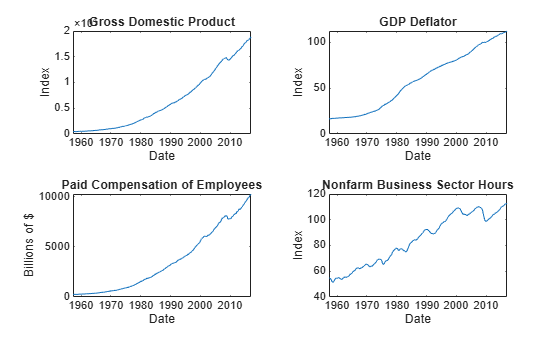figure;
subplot(2,2,1)
plot(FRED.Time,FRED.FEDFUNDS);
title('Federal Funds Rate');
ylabel('Percent');
xlabel('Date');
subplot(2,2,2)
plot(FRED.Time,FRED.PCEC);
title('Consumption Expenditures');
ylabel('Billions of \$');
xlabel('Date');
subplot(2,2,3)
plot(FRED.Time,FRED.GPDI);
title('Gross Private Domestic Investment');
ylabel('Billions of \$');
xlabel('Date');Stabilize all series, except the federal funds rate, by applying the log transform. Scale the resulting series by 100 so that all series are on the same scale.

FRED.GDP = 100*log(FRED.GDP);
FRED.GDPDEF = 100*log(FRED.GDPDEF);
FRED.COE = 100*log(FRED.COE);
FRED.HOANBS = 100*log(FRED.HOANBS);
FRED.PCEC = 100*log(FRED.PCEC);
FRED.GPDI = 100*log(FRED.GPDI);

Create a VEC(1) model using the shorthand syntax. Specify the variable names.

Mdl = vecm(7,4,1);
Mdl.SeriesNames = FRED.Properties.VariableNames
Mdl =
vecm with properties:

Description: "7-Dimensional Rank = 4 VEC(1) Model with Linear Time Trend"
SeriesNames: "GDP"  "GDPDEF"  "COE"  ... and 4 more
NumSeries: 7
Rank: 4
P: 2
Constant: [7×1 vector of NaNs]
Cointegration: [7×4 matrix of NaNs]
Impact: [7×7 matrix of NaNs]
CointegrationConstant: [4×1 vector of NaNs]
CointegrationTrend: [4×1 vector of NaNs]
ShortRun: {7×7 matrix of NaNs} at lag 
Trend: [7×1 vector of NaNs]
Beta: [7×0 matrix]
Covariance: [7×7 matrix of NaNs]

Mdl is a vecm model object. All properties containing NaN values correspond to parameters to be estimated given data.

Estimate the model using the entire data set and the default options.

EstMdl = estimate(Mdl,FRED.Variables)
EstMdl =
vecm with properties:

Description: "7-Dimensional Rank = 4 VEC(1) Model"
SeriesNames: "GDP"  "GDPDEF"  "COE"  ... and 4 more
NumSeries: 7
Rank: 4
P: 2
Constant: [14.1329 8.77841 -7.20359 ... and 4 more]'
Cointegration: [7×4 matrix]
Impact: [7×7 matrix]
CointegrationConstant: [-28.6082 109.555 -77.0912 ... and 1 more]'
CointegrationTrend: [4×1 vector of zeros]
ShortRun: {7×7 matrix} at lag 
Trend: [7×1 vector of zeros]
Beta: [7×0 matrix]
Covariance: [7×7 matrix]

EstMdl is an estimated vecm model object. It is fully specified because all parameters have known values. By default, estimate imposes the constraints of the H1 Johansen VEC model form by removing the cointegrating trend and linear trend terms from the model. Parameter exclusion from estimation is equivalent to imposing equality constraints to zero.

Infer innovations from the estimated model.

E = infer(EstMdl,FRED.Variables);

E is a 238-by-7 matrix of inferred innovations. Columns correspond to the variable names in EstMdl.SeriesNames.

Alternatively, you can return residuals when you call estimate by supplying an output variable in the fourth position.

Plot the residuals on separate plots. Synchronize the residuals with the dates by removing the first EstMdl.P dates.

idx = FRED.Time((EstMdl.P + 1):end);

figure;
subplot(2,2,1)
plot(idx,E(:,1));
title('Residuals: GDP');
ylabel('Index (scaled)');
xlabel('Date');
subplot(2,2,2)
plot(idx,E(:,2));
title('Residuals: GDP Deflator');
ylabel('Index (scaled');
xlabel('Date');
subplot(2,2,3)
plot(idx,E(:,3));
title('Residuals: PCE');
ylabel('Billions of \$ (scaled)');
xlabel('Date');
subplot(2,2,4)
plot(idx,E(:,4));
title('Residuals: NBSH');
ylabel('Index (scaled)');
xlabel('Date');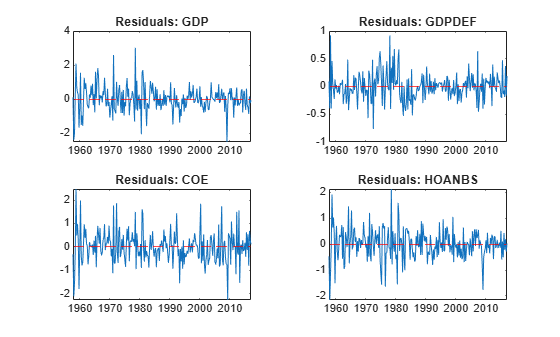figure;
subplot(2,2,1)
plot(idx,E(:,5));
title('Residuals: Federal Funds Rate');
ylabel('Percent');
xlabel('Date');
subplot(2,2,2)
plot(idx,E(:,6));
title('Residuals: COE');
ylabel('Billions of \$ (scaled)');
xlabel('Date');
subplot(2,2,3)
plot(idx,E(:,7));
title('Residuals: GPDI');
ylabel('Billions of \$ (scaled)');
xlabel('Date');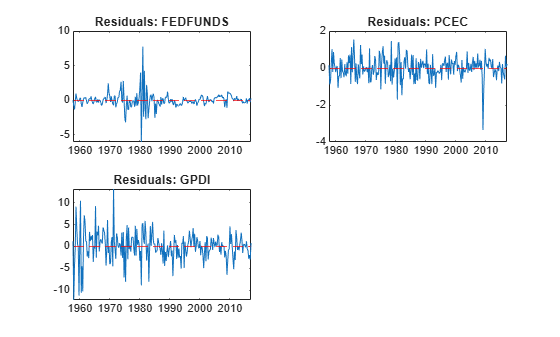The residuals corresponding to the federal funds rate exhibit heteroscedasticity.

Consider the model and data in Infer VEC Model Innovations.

Load the Data_USEconVECModel data set and preprocess the data.

FRED.GDP = 100*log(FRED.GDP);
FRED.GDPDEF = 100*log(FRED.GDPDEF);
FRED.COE = 100*log(FRED.COE);
FRED.HOANBS = 100*log(FRED.HOANBS);
FRED.PCEC = 100*log(FRED.PCEC);
FRED.GPDI = 100*log(FRED.GPDI);

The Data_Recessions data set contains the beginning and ending serial dates of recessions. Load this data set. Convert the matrix of date serial numbers to a datetime array.

dtrec = datetime(Recessions,'ConvertFrom','datenum');

Create a dummy variable that identifies periods in which the U.S. was in a recession or worse. Specifically, the variable should be 1 if FRED.Time occurs during a recession, and 0 otherwise.

isin = @(x)(any(dtrec(:,1) <= x & x <= dtrec(:,2)));
isrecession = double(arrayfun(isin,FRED.Time));

Create a VEC(1) model using the shorthand syntax. Assume that the appropriate cointegration rank is 4. You do not have to specify the presence of a regression component when creating the model. Specify the variable names.

Mdl = vecm(7,4,1);
Mdl.SeriesNames = FRED.Properties.VariableNames;

Estimate the model using the entire sample. Specify the predictor identifying whether the observation was measured during a recession. Return the standard errors.

EstMdl = estimate(Mdl,FRED.Variables,'X',isrecession);

Infer innovations from the estimated model. Supply the predictor data. Return the loglikelihood objective function value.

[E,logL] = infer(EstMdl,FRED.Variables,'X',isrecession);
logL
logL = -1.4656e+03

E is a 238-by-7 matrix of inferred innovations.

Plot the residuals on separate plots. Synchronize the residuals with the dates by removing the first Mdl.P dates.

idx = FRED.Time((EstMdl.P + 1):end);

figure;
subplot(2,2,1)
plot(idx,E(:,1));
title('Residuals: GDP');
ylabel('Index (scaled)');
xlabel('Date');
subplot(2,2,2)
plot(idx,E(:,2));
title('Residuals: GDP Deflator');
ylabel('Index (scaled');
xlabel('Date');
subplot(2,2,3)
plot(idx,E(:,3));
title('Residuals: PCE');
ylabel('Billions of \$ (scaled)');
xlabel('Date');
subplot(2,2,4)
plot(idx,E(:,4));
title('Residuals: NBSH');
ylabel('Index (scaled)');
xlabel('Date');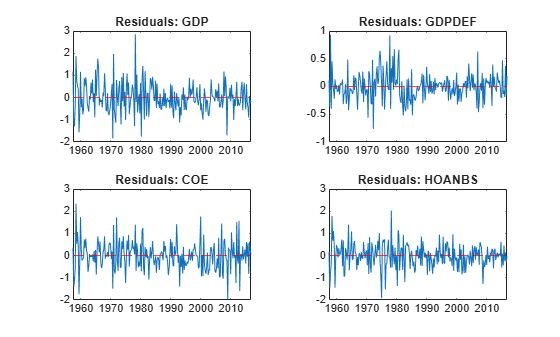figure;
subplot(2,2,1)
plot(idx,E(:,5));
title('Residuals: Federal Funds Rate');
ylabel('Percent');
xlabel('Date');
subplot(2,2,2)
plot(idx,E(:,6));
title('Residuals: COE');
ylabel('Billions of \$ (scaled)');
xlabel('Date');
subplot(2,2,3)
plot(idx,E(:,7));
title('Residuals: GPDI');
ylabel('Billions of \$ (scaled)');
xlabel('Date');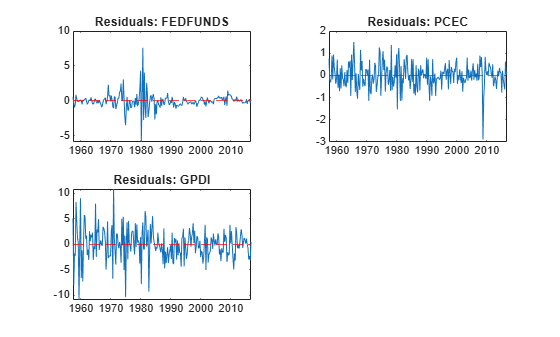The residuals corresponding to the federal funds rate exhibit heteroscedasticity.

Input Arguments

collapse all

VEC model, specified as a vecm model object created by vecm or estimate. Mdl must be fully specified.

Response data, specified as a numobs-by-numseries numeric matrix or a numobs-by-numseries-by-numpaths numeric array.

numobs is the sample size. numseries is the number of response series (Mdl.NumSeries). numpaths is the number of response paths.

Rows correspond to observations, and the last row contains the latest observation. Y represents the continuation of the presample response series in Y0.

Columns must correspond to the response variable names in Mdl.SeriesNames.

Pages correspond to separate, independent numseries-dimensional paths. Among all pages, responses in a particular row occur at the same time.

Data Types: double

Name-Value Arguments

Specify optional comma-separated pairs of Name,Value arguments. Name is the argument name and Value is the corresponding value. Name must appear inside quotes. You can specify several name and value pair arguments in any order as Name1,Value1,...,NameN,ValueN.

Example: 'Y0',Y0,'X',X uses the matrix Y0 as presample responses and the matrix X as predictor data in the regression component.

Presample responses providing initial values for the model, specified as the comma-separated pair consisting of 'Y0' and a numpreobs-by-numseries numeric matrix or a numpreobs-by-numseries-by-numprepaths numeric array.

numpreobs is the number of presample observations. numprepaths is the number of presample response paths.

Rows correspond to presample observations, and the last row contains the latest presample observation. Y0 must have at least Mdl.P rows. If you supply more rows than necessary, infer uses only the latest Mdl.P observations.

Columns must correspond to the columns of Y.

Pages correspond to separate independent paths.

• If Y0 is a matrix, then infer applies it to each path (page) in Y. Therefore, all paths in Y derive from common initial conditions.

• Otherwise, infer applies Y0(:,:,j) to Y(:,:,j). Y0 must have at least numpaths pages, and infer uses only the first numpaths pages.

Among all pages, observations in a particular row occur at the same time.

By default, infer uses Y(1:Mdl.P,:) as presample observations. This action reduces the effective sample size.

Data Types: double

Predictor data for the regression component in the model, specified as the comma-separated pair consisting of 'X' and a numeric matrix containing numpreds columns.

numpreds is the number of predictor variables (size(Mdl.Beta,2)).

Rows correspond to observations, and the last row contains the latest observation. infer does not use the regression component in the presample period. Therefore, X must have at least as many observations as are used after the presample period.

• If you specify Y0, then X must have at least numobs rows (see Y).

• Otherwise, X must have at least numobsMdl.P observations to account for the presample removal.

In either case, if you supply more rows than necessary, infer uses the latest observations only.

Columns correspond to individual predictor variables. All predictor variables are present in the regression component of each response equation.

infer applies X to each path (page) in Y; that is, X represents one path of observed predictors.

By default, infer excludes the regression component, regardless of its presence in Mdl.

Data Types: double

Note

NaN values in Y, Y0, and X indicate missing values. infer removes missing values from the data by list-wise deletion.

1. If Y is a 3-D array, then infer horizontally concatenates the pages of Y to form a numobs-by-(numpaths*numseries + numpreds) matrix.

2. If a regression component is present, then infer horizontally concatenates X to Y to form a numobs-by-numpaths*numseries + 1 matrix. infer assumes that the last rows of each series occur at the same time.

3. infer removes any row that contains at least one NaN from the concatenated data.

4. infer applies steps 1 and 3 to the presample paths in Y0.

This process ensures that the inferred output innovations of each path are the same size and are based on the same observation times. In the case of missing observations, the results obtained from multiple paths of Y can differ from the results obtained from each path individually.

This type of data reduction reduces the effective sample size.

Output Arguments

collapse all

Inferred multivariate innovations series, returned as either a numeric matrix, or as a numeric array that contains columns and pages corresponding to Y.

• If you specify Y0, then E has numobs rows (see Y).

• Otherwise, E has numobsMdl.P rows to account for the presample removal.

Loglikelihood objective function value associated with the VEC model Mdl, returned as a numeric scalar or a numpaths-element numeric vector. logL(j) corresponds to the response path in Y(:,:,j).

Algorithms

• infer infers innovations by evaluating the VEC model Mdl with respect to the innovations using the supplied data Y, Y0, and X. The inferred innovations are

${\stackrel{^}{\epsilon }}_{t}=\stackrel{^}{\Phi }\left(L\right)\Delta {y}_{t}-\stackrel{^}{A}{\stackrel{^}{B}}^{\prime }{y}_{t-1}-\stackrel{^}{c}-\stackrel{^}{d}t-\stackrel{^}{\beta }{x}_{t}.$

• infer uses this process to determine the time origin t0 of models that include linear time trends.

• If you do not specify Y0, then t0 = 0.

• Otherwise, infer sets t0 to size(Y0,1)Mdl.P. Therefore, the times in the trend component are t = t0 + 1, t0 + 2,..., t0 + numobs, where numobs is the effective sample size (size(Y,1) after infer removes missing values). This convention is consistent with the default behavior of model estimation in which estimate removes the first Mdl.P responses, reducing the effective sample size. Although infer explicitly uses the first Mdl.P presample responses in Y0 to initialize the model, the total number of observations in Y0 and Y (excluding missing values) determines t0.

 Hamilton, James D. Time Series Analysis. Princeton, NJ: Princeton University Press, 1994.

 Johansen, S. Likelihood-Based Inference in Cointegrated Vector Autoregressive Models. Oxford: Oxford University Press, 1995.

 Juselius, K. The Cointegrated VAR Model. Oxford: Oxford University Press, 2006.

 Lütkepohl, H. New Introduction to Multiple Time Series Analysis. Berlin: Springer, 2005.